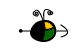Determining Molar Mass

We can use a measurement of any one of the following properties to determine the molar mass (molecular weight) of an unknown that is the solute in a solution:

From Boiling Point Elevation

1. Determine the change in boiling point from the observed boiling point of the solution and the boiling point of the pure solvent.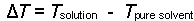2. Determine the molal concentration, m, from the change in boiling point and the boiling point elevation constant.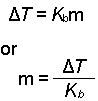3. Determine the moles of unknown (the solute) from the molality of the solution and the mass of solvent (in kilograms) used to make the solution.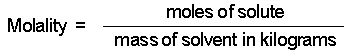4. Determine the molar mass from the mass of the unknown and the number of moles of unknown.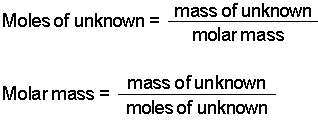Top

From Freezing Point Depression

1. Determine the change in freezing point from the observed freezing point of the solution and the freezing point of the pure solvent. (This will be a negative number.)2. Determine the molal concentration, m, from the change in freezing point and the freezing point depression constant.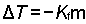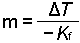3. Determine the moles of unknown (the solute) from the molality of the solution and the mass of solvent (in kilograms) used to make the solution.4. Determine the molar mass from the mass of the unknown and the number of moles of unknown.Top

From Osmotic Pressure

1. Determine the molar concentration of the unknown in the solution from the observed osmotic pressure.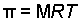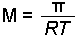2. Determine the moles of unknown (the solute) from the molarity of the solution and the volume (in liters) of the solution.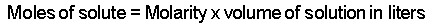3. Determine the molar mass from the mass of the unknown and the number of moles of unknown.Top